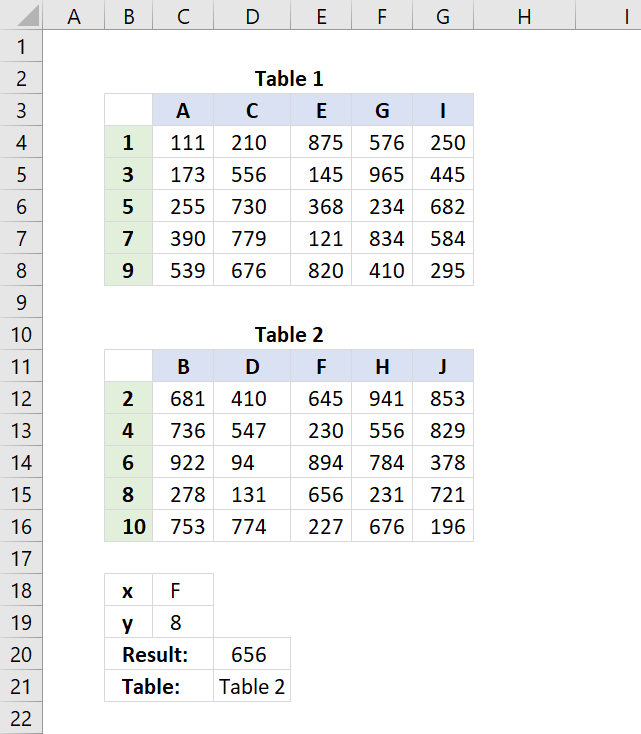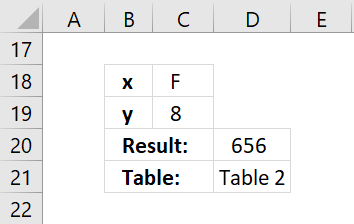Author: Oscar Cronquist Article last updated on March 25, 2018The following formula performs a two-way lookup in two different tables.

Formula in cell D20:

=IFERROR(INDEX(C4:G8, MATCH(C19, B4:B8, 0), MATCH(C18, C3:G3, 0)), INDEX(C12:G16, MATCH(C19, B12:B16, 0), MATCH(C18, C11:G11, 0)))

If the value is not found in table 1 the formula continues to table 2. If nothing is found in table 2 as well the formula returns #N/A.

The formula does not return multiple values from different tables, in that case, check out this User defined Function:

Two-way lookup using multiple tables [UDF]

This article describes a User Defined Function that lookups values in multiple cross reference tables based on two conditions. A […]

Formula in cell D21:

=CHOOSE(IF(ISERROR(MATCH(C18,C3:G3,0)),2,1),"Table 1", "Table 2")

The formula above checks if the value is found in table 1 or table 2.

### Explaining formula in cell D20

#### Step 1 - Find relative position of y value in table 1

The MATCH function looks for number 8 in the vertical cell range B4:B8.

MATCH(C19,B4:B8,0)

becomes

MATCH(8,{1;3;5;7;9},0)

#### Step 2 - Find relative position of x value in table 1

The second MATCH function looks for "F" in the horizontal cell range C3:G3.

MATCH(C18,C3:G3,0)

becomes

MATCH("F",{"A","C","E","G","I"},0)

#### Step 3 - Get value based on coordinates in table 1

INDEX(C4:G8,MATCH(C19,B4:B8,0),MATCH(C18,C3:G3,0))

becomes

INDEX(C4:G8,#N/A,#N/A)

and returns #N/A. The value is not found in the first table.

#### Step 4 - IF error continue to table 2 and repeat

=IFERROR(INDEX(C4:G8, MATCH(C19, B4:B8, 0), MATCH(C18, C3:G3, 0)), INDEX(C12:G16, MATCH(C19, B12:B16, 0), MATCH(C18, C11:G11, 0)))

becomes

=IFERROR(#N/A,INDEX(C12:G16,MATCH(C19,B12:B16,0),MATCH(C18,C11:G11,0)))

becomes

=IFERROR(#N/A,INDEX(C12:G16,4,3))

becomes

=IFERROR(#N/A,656)

and returns 656 in cell D20.### Multiple tables

It is possible to use more than two tables, simply use this template:

IFERROR(first_formula,IFERROR(second_formula,third_formula))

The formula would then be:

=IFERROR(INDEX(C4:G8, MATCH(C19, B4:B8, 0), MATCH(C18, C3:G3, 0)), IFERROR(INDEX(C12:G16, MATCH(C19, B12:B16, 0), MATCH(C18, C11:G11, 0)),INDEX(C20:G24, MATCH(C19, B20:B24, 0), MATCH(C18, C19:G19, 0)))